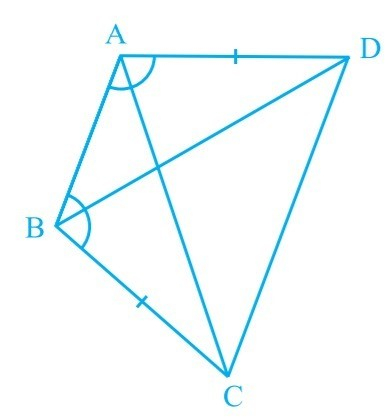(i) $\triangle A B D \cong \triangle B A C$
(ii) $B D=A C$
(iii) $\angle ABD=\angle BAC$ "
">

# ABCD is a quadrilateral in which $A D=B C$ and $\angle D A B=\angle C B A$. Prove that.(i) $\triangle A B D \cong \triangle B A C$(ii) $B D=A C$(iii) $\angle ABD=\angle BAC$ "

Given:
ABCD is a quatrilateral in which $A D=B C$ and $\angle D A B=\angle C B A$.
To do:
We have to prove that,
(i) $\vartriangle ABD \cong\ BAC$
(ii) $BD=AC$
(iii) $\angle ABD=\angle BAC$
Solution:
In $\vartriangle ABD$ and $\vartriangle BAC$,
$AD=BC$ (Given)
$\angle DAB=\angle CBA$ (Given)
$AB=BA$ (Common)
Therefore,
$\vartriangle ABD \cong\ BAC$ (By SAS congruence rule)
We know that,
Corresponding parts of congruent triangles are equal.
Therefore,
$BD=AC$ (By CPCT)
$\angle ABD=\angle BAC$ (By CPCT)
Hence proved.

Updated on: 10-Oct-2022

48 Views